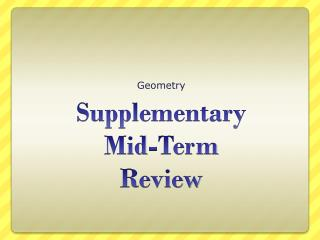DownloadDownload PresentationSupplementary Mid-Term Review

# Supplementary Mid-Term Review

Télécharger la présentation## Supplementary Mid-Term Review

- - - - - - - - - - - - - - - - - - - - - - - - - - - E N D - - - - - - - - - - - - - - - - - - - - - - - - - - -
##### Presentation Transcript

1. Geometry SupplementaryMid-TermReview

2. Find the area of the given rectangle A. C. B. D.

3. A plane has ____ dimensions. • 0 • 1 • 2 • 3 • ∞

4. A plane is determined by: • 2 collinear points • 2 noncollinear points • a line • 3 collinear points • 3 noncollinear points

5. True or False?? Point B is between points A and D. D B FALSE A

6. Translate (3, – 2) according to the rule (x, y)  (x – 3, y +8) • (6, 6) • (0, 6) • (0, -10) • (6, -10)

7. What kind of triangle has side lengths of 3, 4, 5? • Not a triangle • Acute triangle • Right triangle • Obtuse triangle

8. What kind of triangle has side lengths of 3, 4, 6? • Not a triangle • Acute triangle • Right triangle • Obtuse triangle

9. What kind of triangle has side lengths of 3, 4, 9? • Not a triangle • Acute triangle • Right triangle • Obtuse triangle

10. What kind of triangle has angle measures of 30˚, 40˚, 90˚? • Not a triangle • Acute triangle • Right triangle • Obtuse triangle

11. If M is the centroid of ∆DOG, and MP = 10, find MO. • 10 • 20 • 25 • 30 D . P M O G

12. If 1 and 2 are a linear pair and 1 = 30˚, find the measure of 2. • 30˚ • 60˚ • 150˚ • 170˚

13. Find the complement of an angle with a measure of 50˚ • 40˚ • 50˚ • 130˚ • 150˚

14. What is the midpoint of the segment with endpoints (4, – 6) and (5, 12)? (-4.5, 3) (4.5, 3) (9, 6) (4.5, -3) (4.5, 6)